## Neutralization Reaction and Net Ionic Equations for Neutralization Reactions

### Learning Objectives

• Define neutralizations reaction.
• Write balanced equations for neutralization reactions.
• Write net ionic equations for neutralization reactions.

### Who cleans up afterwards?

Pouring concrete and working it are messy jobs. In the process, a lot of wastewater with an alkaline pH is generated. Often, regulations require that this wastewater be cleaned up at the site. One practical way to neutralize the basic pH is to bubble CO2 into the water. The carbon dioxide forms a weak acid (carbonic acid, H2CO3) in solution which serves to bring the alkaline pH down to something closer to neutral.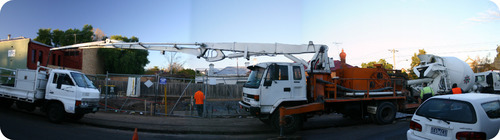## Neutralization Reactions and Net Ionic Equations for Neutralization Reactions

A neutralization reaction is a reaction in which an acid and a base react in an aqueous solution to produce a salt and water. The aqueous sodium chloride that is produced in the reaction is called a salt. A salt is an ionic compound composed of a cation from a base and an anion from an acid. A salt is essentially any ionic compound that is neither an acid nor a base.

### Strong Acid-Strong Base Reactions

When equal amounts of a strong acid such as hydrochloric acid are mixed with a strong base such as sodium hydroxide, the result is a neutral solution. The products of the reaction do not have the characteristics of either an acid or a base. Here is the balanced molecular equation.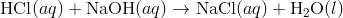Chemical reactions occurring in aqueous solution are more accurately represented with a net ionic equation. The full ionic equation for the neutralization of hydrochloric acid by sodium hydroxide is written as follows: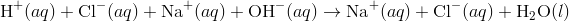Since the acid and base are both strong, they are fully ionized and so are written as ions, as is the NaCl formed as a product. The sodium and chloride ions are spectator ions in the reaction, leaving the following as the net ionic reaction.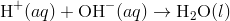All neutralization reactions of a strong acid with a strong base simplify to the net ionic reaction of hydrogen ion combining with hydroxide ion to produce water.

What if the acid is a diprotic acid such as sulfuric acid? The balanced molecular equation now involves a 1:2 ratio between acid and base.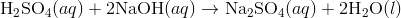In order for the reaction to be a full neutralization, twice as many moles of NaOH must react with the H2SO4. The sodium sulfate salt is soluble, and so the net ionic reaction is again the same. Different mole ratios occur for other polyprotic acids or bases with multiple hydroxides such as Ca(OH)2.

### Reactions Involving a Weak Acid or Weak Base

Reactions where at least one of the components is weak do not generally result in a neutral solution. The reaction between weak nitrous acid and strong potassium hydroxide is shown below.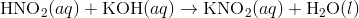In order to write the net ionic equation, the weak acid must be written as a molecule since it does not ionize to a great extent in water. The base and the salt are fully dissociated.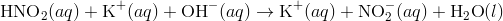The only spectator ion is the potassium ion, resulting in the net ionic equation: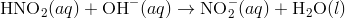The strong hydroxide ion essentially “forces” the weak nitrous acid to become ionized. The hydrogen ion from the acid combines with the hydroxide ion to form water, leaving the nitrite ion as the other product. The resulting solution is not neutral (pH = 7), but instead is slightly basic.

Reactions can also involve a weak base and strong acid, resulting in a solution that is slightly acidic. The molecular and net ionic equations for the reaction of hydrochloric acid and ammonia are shown below.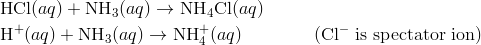Reactions between acids and bases that are both weak may result in solutions that are neutral, acidic, or basic.

### Summary

• Neutralization is defined.
• Equations for acid-base neutralizations are given.
• Net ionic equations for neutralization reactions are given.

### Practice

Do the problems on the sheet at the link below:

http://www.bbc.co.uk/bitesize/standard/chemistry/acids/reactions/revision/4/

### Review

1. What is a neutralization reaction?
2. What is a salt?
3. Write the net ionic equation for the neutralization of a strong acid and a strong base.

### Glossary

• neutralization reaction: A reaction in which an acid and a base react in an aqueous solution to produce a salt and water.
• salt: An ionic compound composed of a cation from a base and an anion from an acid.